## 奇瑞捷豹路虎 捷豹XFL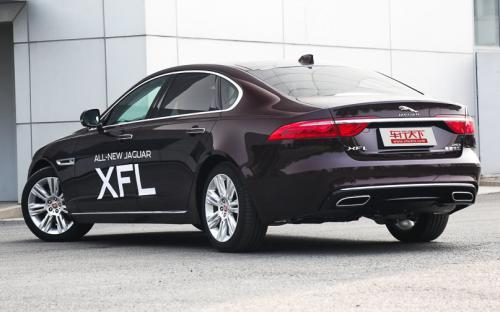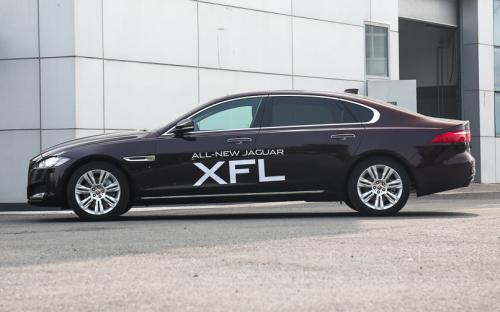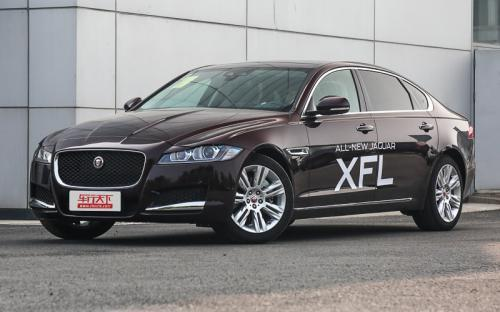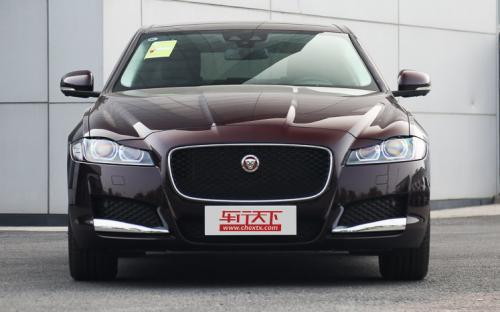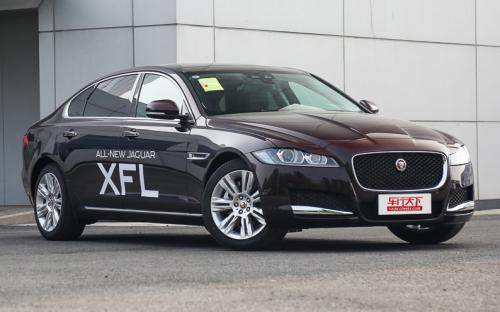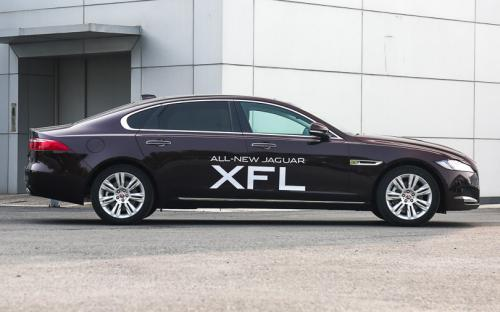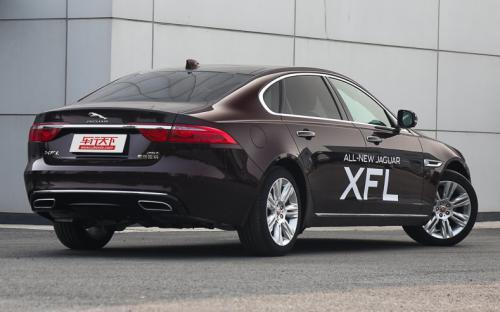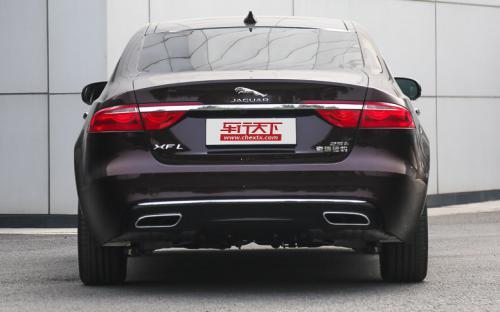0 种颜色可选2017款最低售价：38.80 万元起

5093(mm)1880(mm)1456(mm)##### 配置亮点：
• 胎压监测装置

• ISOFIX儿童座椅接口

• 车身稳定控制(ESC/ESP/DSC等)

• 电动天窗

• 定速巡航

• 后倒车雷达

• 真皮座椅

• GPS导航系统

• 氙气大灯

• 后视镜加热

2017款 XFL 2.0T 240PS 尊享版 (238张)
• 2017款 XFL 2.0T 240PS 尊享版 (238张)
• 2017款 XFL 3.0T 340PS 奢华版 (291张)
• 2017款 XFL 2.0T 200PS 精英版 (143张)奇瑞捷豹路虎 捷豹XFL 在售车型

排量 车型 厂商指导价 本地最低报价 购车工具
2.0L XFL 2.0T 200PS 风华版 停产在售 8挡手自一体 38.80万 询底价+对比
XFL 2.0T 200PS 精英版 停产在售 8挡手自一体 40.80万 询底价+对比
XFL 2.0T 200PS 豪华版 停产在售 8挡手自一体 43.60万 询底价+对比
XFL 2.0T 240PS 豪华版 停产在售 8挡手自一体 45.60万 询底价+对比
XFL 2.0T 240PS 尊享版 停产在售 8挡手自一体 48.80万 询底价+对比
XFL 2.0T 240PS 奢华版 停产在售 8挡手自一体 56.80万 询底价+对比
XFL 3.0T 340PS 尊享版 停产在售 8挡手自一体 61.80万 询底价+对比
XFL 3.0T 340PS 奢华版 停产在售 8挡手自一体 68.80万 询底价+对比
3.0L XFL 2.0T 200PS 风华版 停产在售 8挡手自一体 38.80万 询底价+对比
XFL 2.0T 200PS 精英版 停产在售 8挡手自一体 40.80万 询底价+对比
XFL 2.0T 200PS 豪华版 停产在售 8挡手自一体 43.60万 询底价+对比
XFL 2.0T 240PS 豪华版 停产在售 8挡手自一体 45.60万 询底价+对比
XFL 2.0T 240PS 尊享版 停产在售 8挡手自一体 48.80万 询底价+对比
XFL 2.0T 240PS 奢华版 停产在售 8挡手自一体 56.80万 询底价+对比
XFL 3.0T 340PS 尊享版 停产在售 8挡手自一体 61.80万 询底价+对比
XFL 3.0T 340PS 奢华版 停产在售 8挡手自一体 68.80万 询底价+对比

奇瑞捷豹路虎 捷豹XFL 经销商

查看更多 >>
更多 >>

本地降价排名

### 奇瑞捷豹路虎 捷豹XFL 动力加速

捷豹XFL 0-100公里加速时间分布在 6.0-8.1秒 属于 高性能级

动力级别 加速时间 车型
运动级(2款) 8.1s XFL 2.0T 200PS 风华版XFL 2.0T 200PS 精英版XFL 2.0T 200PS 豪华版
7.4s XFL 2.0T 240PS 豪华版XFL 2.0T 240PS 尊享版XFL 2.0T 240PS 奢华版
高性能级(1款) 6.0s XFL 3.0T 340PS 尊享版XFL 3.0T 340PS 奢华版

奇瑞捷豹路虎 捷豹XFL 视频

奇瑞捷豹路虎 捷豹XFL 新闻资讯

# 国产捷豹XFL正式上市 售价38.8万元起

上市新车 超过10341次关注

2016年8月29日，国产捷豹XFL正式上市发售，据悉，捷豹XFL在分别搭载2.0T高低功率版本涡轮增压发动机及3.0SCV6机械增压发动机基础上根据配置的差异化共推出8款车型，...

# 三种动力选择 捷豹XFL将于8月29日上市

即将上市 超过9302次关注

据官方获悉，国产捷豹XFL将于8月29日正式上市，新车上市后将提供三种动力选择，包括两种调校的2.0T发动机，以及3.0TV6机械增压发动机。

# 2016 CES 捷豹推全新车载互联系统

行业动态 超过8848次关注

在2016CES展上，捷豹携手英特尔带来了全新的车载互联系统，根据现场得到的资料，其或将率先搭载在全新SUVF-PACE。未来将会有越来越多的捷豹以及路虎的车型搭载触摸...

猜你喜欢

﻿
• 快速找车
• 选择品牌
• 选择品牌
• A  奥迪
• A  阿斯顿·马丁
• A  阿尔法·罗密欧
• B  宝沃
• B  布加迪
• B  巴博斯
• B  保时捷
• B  宾利
• B  奔驰
• B  宝马
• B  本田
• B  别克
• B  标致
• B  比亚迪
• B  宝骏
• B  北汽制造
• B  北汽新能源
• B  北汽幻速
• B  北汽威旺
• B  北京汽车
• B  奔腾
• B  北汽绅宝
• C  长安
• C  长安商用
• C  长城
• C  昌河
• D  大众
• D  道奇
• D  DS
• D  东南
• D  东风风神
• D  东风风行
• D  东风小康
• D  东风风度
• D  东风
• F  福特
• F  丰田
• F  菲亚特
• F  法拉利
• F  福田
• F  福迪
• F  福汽启腾
• G  观致
• G  广汽传祺
• G  广汽吉奥
• G  GMC
• H  红旗
• H  汉腾汽车
• H  哈弗
• H  哈飞
• H  海格
• H  海马
• H  华颂
• H  黄海
• H  华泰
• H  恒天
• J  吉利汽车
• J  捷豹
• J  Jeep
• J  江淮
• J  江铃
• J  金杯
• J  九龙
• J  金旅
• K  凯翼
• K  凯迪拉克
• K  克莱斯勒
• K  科尼塞克
• K  卡威
• K  开瑞
• L  路虎
• L  林肯
• L  劳斯莱斯
• L  兰博基尼
• L  雷克萨斯
• L  铃木
• L  雷诺
• L  理念
• L  力帆
• L  莲花汽车
• L  猎豹
• L  路特斯
• L  陆风
• M  马自达
• M  MG
• M  MINI
• M  玛莎拉蒂
• M  摩根
• M  迈凯轮
• N  纳智捷
• O  欧宝
• O  讴歌
• O  欧朗
• Q  奇瑞
• Q  起亚
• Q  启辰
• R  日产
• R  荣威
• R  瑞麒
• S  三菱
• S  斯威汽车
• S  萨博
• S  smart
• S  斯柯达
• S  斯巴鲁
• S  思铭
• S  双龙
• S  上汽大通
• S  双环
• T  特斯拉
• T  腾势
• W  沃尔沃
• W  五菱汽车
• W  五十铃
• W  威兹曼
• W  威麟
• X  现代
• X  雪佛兰
• X  雪铁龙
• X  西雅特
• Y  一汽
• Y  英菲尼迪
• Y  英致
• Y  依维柯
• Y  野马汽车
• Y  永源
• Z  众泰
• Z  中华
• Z  中兴
• Z  知豆
• 选择车系
• 选择车系
• 车型对比
• 选择品牌
• 选择品牌
• A  奥迪
• A  阿斯顿·马丁
• A  阿尔法·罗密欧
• B  宝沃
• B  布加迪
• B  巴博斯
• B  保时捷
• B  宾利
• B  奔驰
• B  宝马
• B  本田
• B  别克
• B  标致
• B  比亚迪
• B  宝骏
• B  北汽制造
• B  北汽新能源
• B  北汽幻速
• B  北汽威旺
• B  北京汽车
• B  奔腾
• B  北汽绅宝
• C  长安
• C  长安商用
• C  长城
• C  昌河
• D  大众
• D  道奇
• D  DS
• D  东南
• D  东风风神
• D  东风风行
• D  东风小康
• D  东风风度
• D  东风
• F  福特
• F  丰田
• F  菲亚特
• F  法拉利
• F  福田
• F  福迪
• F  福汽启腾
• G  观致
• G  广汽传祺
• G  广汽吉奥
• G  GMC
• H  红旗
• H  汉腾汽车
• H  哈弗
• H  哈飞
• H  海格
• H  海马
• H  华颂
• H  黄海
• H  华泰
• H  恒天
• J  吉利汽车
• J  捷豹
• J  Jeep
• J  江淮
• J  江铃
• J  金杯
• J  九龙
• J  金旅
• K  凯翼
• K  凯迪拉克
• K  克莱斯勒
• K  科尼塞克
• K  卡威
• K  开瑞
• L  路虎
• L  林肯
• L  劳斯莱斯
• L  兰博基尼
• L  雷克萨斯
• L  铃木
• L  雷诺
• L  理念
• L  力帆
• L  莲花汽车
• L  猎豹
• L  路特斯
• L  陆风
• M  马自达
• M  MG
• M  MINI
• M  玛莎拉蒂
• M  摩根
• M  迈凯轮
• N  纳智捷
• O  欧宝
• O  讴歌
• O  欧朗
• Q  奇瑞
• Q  起亚
• Q  启辰
• R  日产
• R  荣威
• R  瑞麒
• S  三菱
• S  斯威汽车
• S  萨博
• S  smart
• S  斯柯达
• S  斯巴鲁
• S  思铭
• S  双龙
• S  上汽大通
• S  双环
• T  特斯拉
• T  腾势
• W  沃尔沃
• W  五菱汽车
• W  五十铃
• W  威兹曼
• W  威麟
• X  现代
• X  雪佛兰
• X  雪铁龙
• X  西雅特
• Y  一汽
• Y  英菲尼迪
• Y  英致
• Y  依维柯
• Y  野马汽车
• Y  永源
• Z  众泰
• Z  中华
• Z  中兴
• Z  知豆
• 选择车系
• 选择车系
• 选择车型
• 选择车型
• 意见反馈